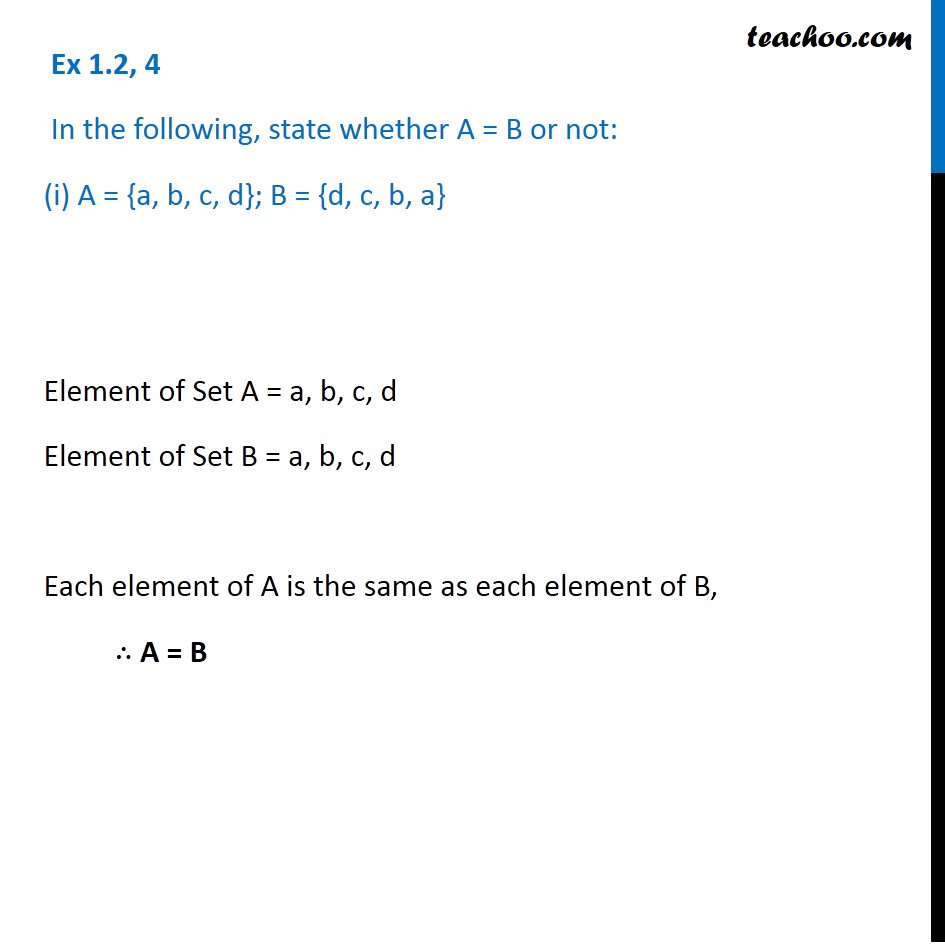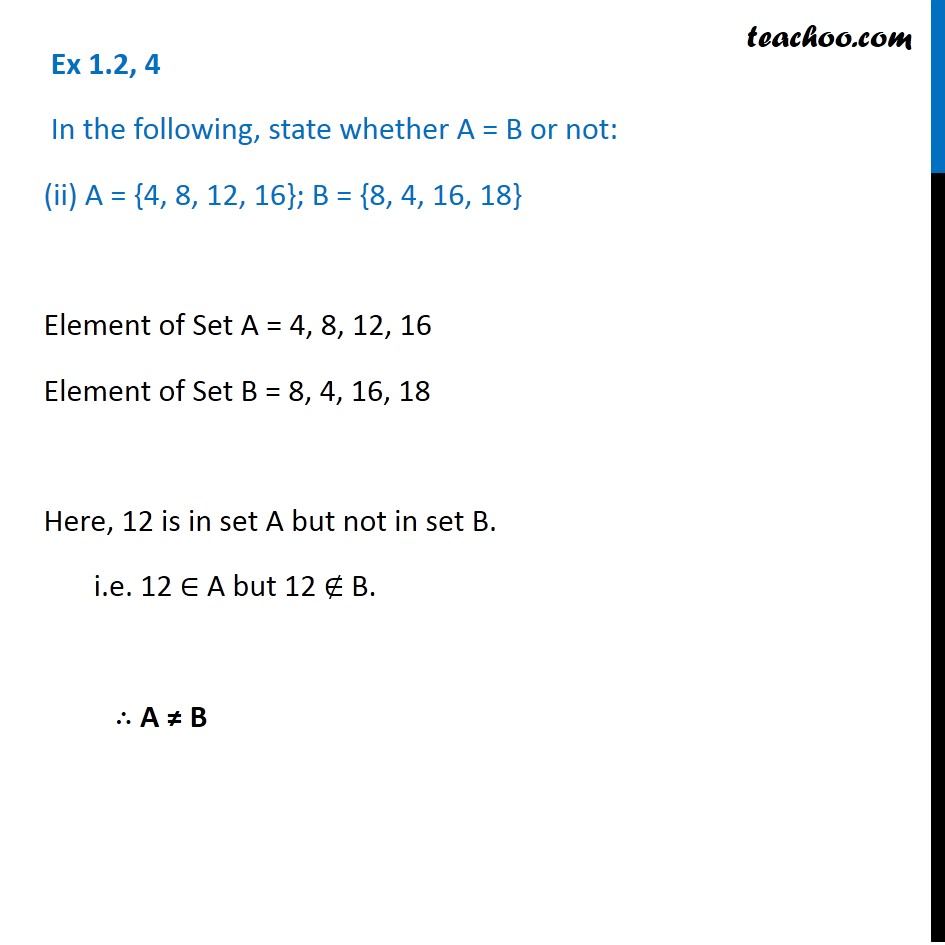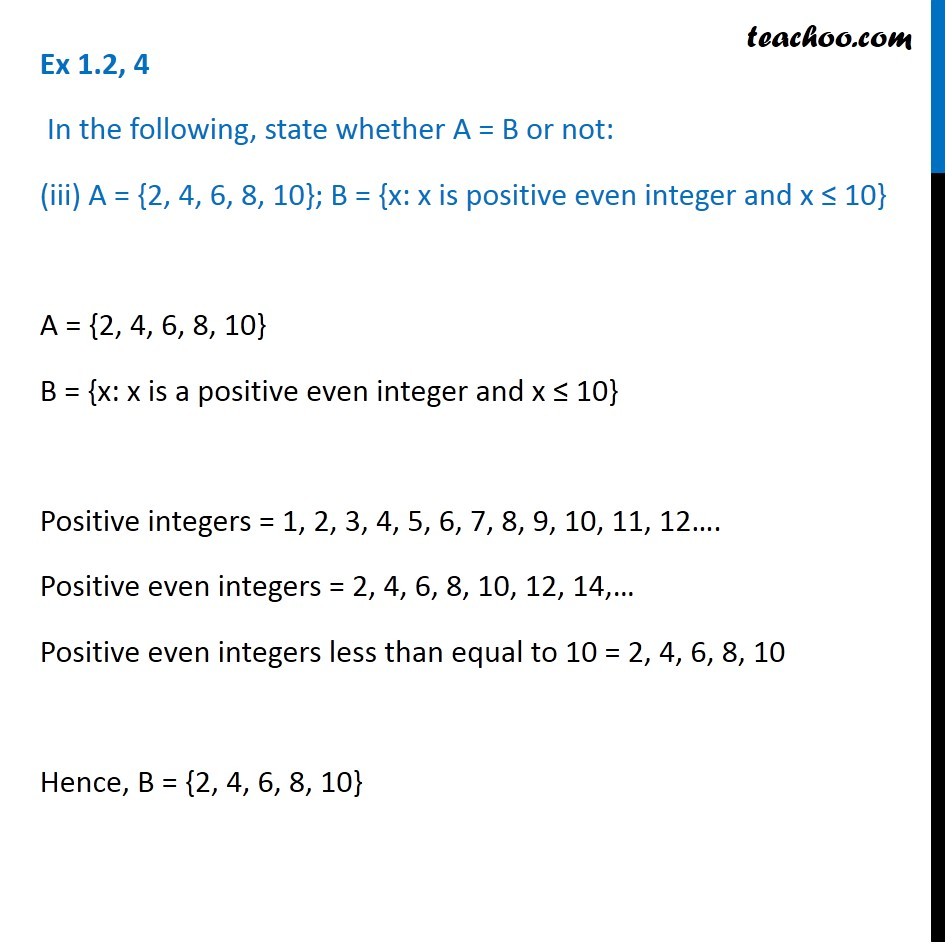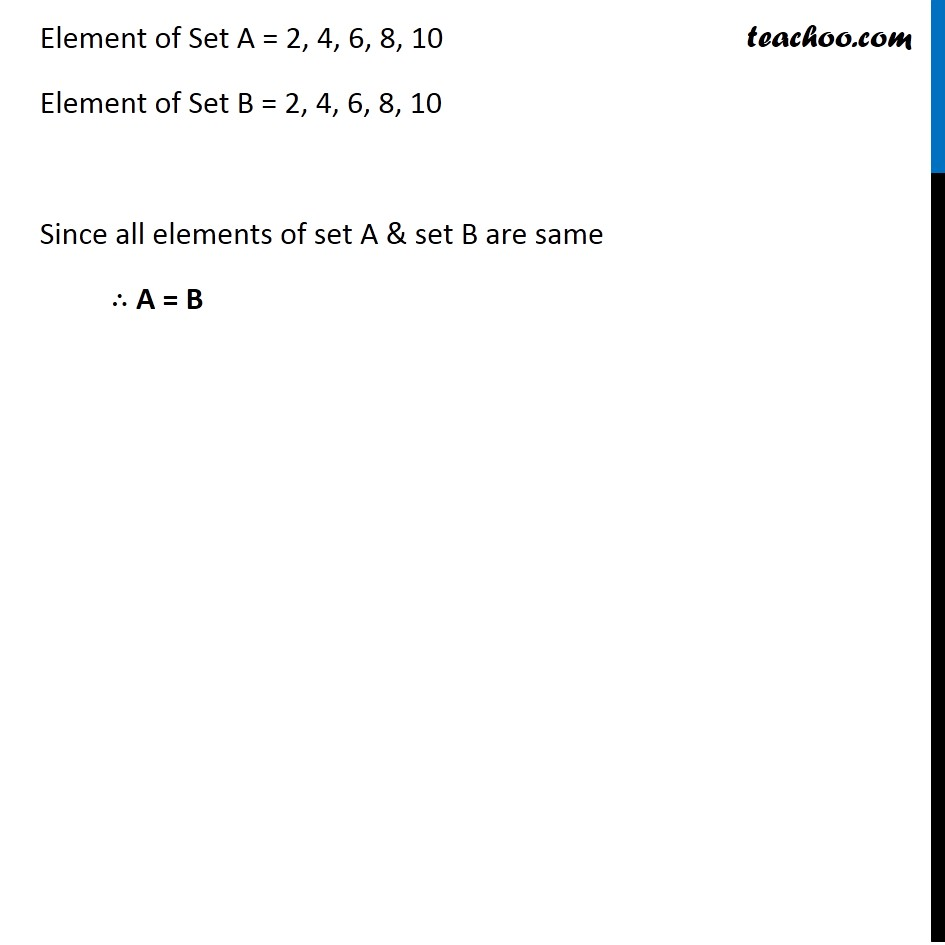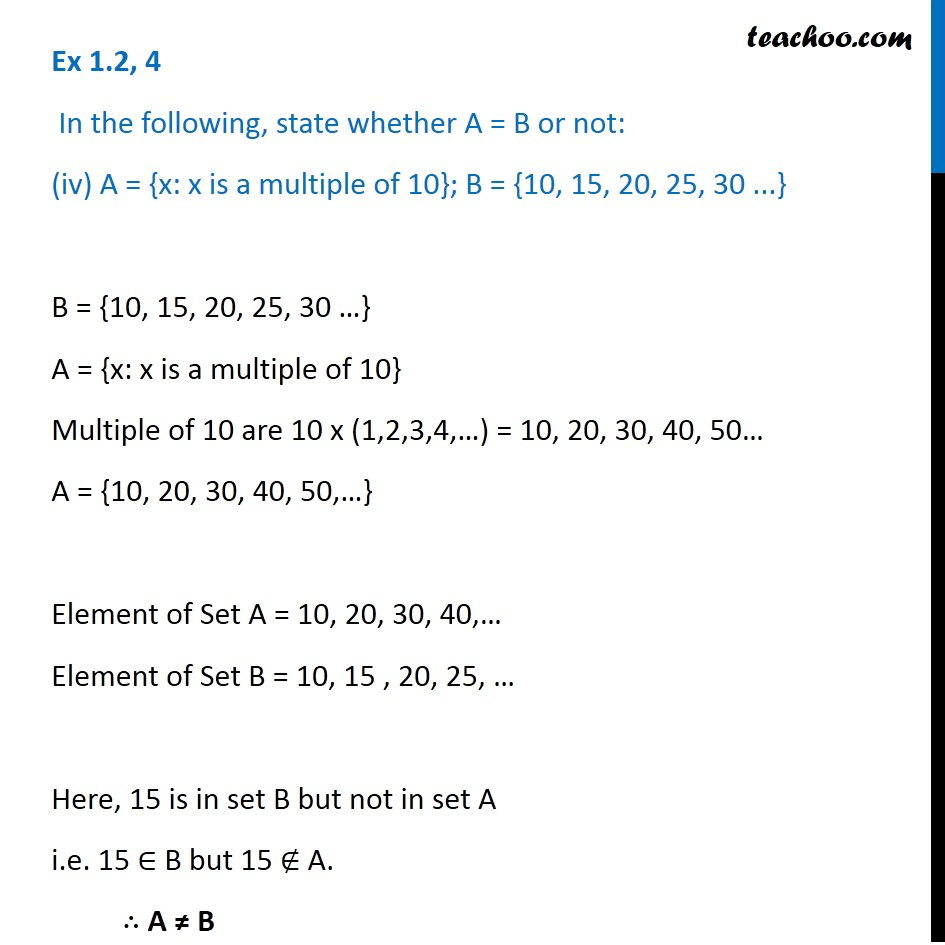1. Chapter 1 Class 11 Sets
2. Serial order wise
3. Ex 1.2

Transcript

Ex 1.2, 4 In the following, state whether A = B or not: (i) A = {a, b, c, d}; B = {d, c, b, a} Element of Set A = a, b, c, d Element of Set B = a, b, c, d Each element of A is the same as each element of B, ∴ A = B Ex 1.2, 4 In the following, state whether A = B or not: (iii) A = {2, 4, 6, 8, 10}; B = {x: x is positive even integer and x ≤ 10} A = {2, 4, 6, 8, 10} B = {x: x is a positive even integer and x ≤ 10} Positive integers = 1, 2, 3, 4, 5, 6, 7, 8, 9, 10, 11, 12…. Positive even integers = 2, 4, 6, 8, 10, 12, 14,… Positive even integers less than equal to 10 = 2, 4, 6, 8, 10 Hence, B = {2, 4, 6, 8, 10} Element of Set A = 2, 4, 6, 8, 10 Element of Set B = 2, 4, 6, 8, 10 Since all elements of set A & set B are same ∴ A = B Ex 1.2, 4 In the following, state whether A = B or not: (iv) A = {x: x is a multiple of 10}; B = {10, 15, 20, 25, 30 ...} B = {10, 15, 20, 25, 30 …} A = {x: x is a multiple of 10} Multiple of 10 are 10 x (1,2,3,4,…) = 10, 20, 30, 40, 50… A = {10, 20, 30, 40, 50,…} Element of Set A = 10, 20, 30, 40,… Element of Set B = 10, 15 , 20, 25, … Here, 15 is in set B but not in set A i.e. 15 ∈ B but 15 ∉ A. ∴ A ≠ B

Ex 1.2

Chapter 1 Class 11 Sets
Serial order wise